• 互相关函数的关系
千次阅读
2021-04-22 00:43:02

1. 首先说说自相关和互相关的概念。

这个是信号分析里的概念，他们分别表示的是两个时间序列之间和同一个时间序列在任意两个不同时刻的取值之间的相关程度，即互相关函数是描述随机信号x(t),y(t)在任意两个不同时刻t1，t2的取值之间的相关程度，自相关函数是描述随机信号x(t)在任意两个不同时刻t1，t2的取值之间的相关程度。

自相关函数是描述随机信号X(t)在任意两个不同时刻t1，t2的取值之间的相关程度；互相关函数给出了在频域内两个信号是否相关的一个

判断指标，把两测点之间信号的互谱与各自的自谱联系了起来。它能用来确定输出信号有多大程度来自输入信号，对修正测量中接入噪声源而产生

的误差非常有效.

事实上，在图象处理中，自相关和互相关函数的定义如下：设原函数是f(t)，则自相关函数定义为R(u)=f(t)*f(-t)，其中*表示卷积；设

两个函数分别是f(t)和g(t)，则互相关函数定义为R(u)=f(t)*g(-t)，它反映的是两个函数在不同的相对位置上互相匹配的程度。

那么，如何在matlab中实现这两个相关并用图像显示出来呢？

dt=.1;

t=[0:dt:100];

x=cos(t);

[a,b]=xcorr(x,'unbiased');

plot(b*dt,a)

上面代码是求自相关函数并作图，对于互相关函数，稍微修改一下就可以了，即把[a,b]=xcorr(x,'unbiased');改为[a,b]=xcorr

(x,y,'unbiased');便可。

2. 实现过程：

在Matalb中，求解xcorr的过程事实上是利用Fourier变换中的卷积定理进行的，即R(u)=ifft(fft(f)×fft(g))，其中×表示乘法，注：此

公式仅表示形式计算，并非实际计算所用的公式。当然也可以直接采用卷积进行计算，但是结果会与xcorr的不同。事实上，两者既然有定理保证

，那么结果一定是相同的，只是没有用对公式而已。下面是检验两者结果相同的代码：

dt=.1;

t=[0:dt:100];

x=3*sin(t);

y=cos(3*t);

subplot(3,1,1);

plot(t,x);

subplot(3,1,2);

plot(t,y);

[a,b]=xcorr(x,y);

subplot(3,1,3);

plot(b*dt,a);

yy=cos(3*fliplr(t)); % or use: yy=fliplr(y);

z=conv(x,yy);

pause;

subplot(3,1,3);

plot(b*dt,z,'r');

即在xcorr中不使用scaling。

3. 其他相关问题：

1) 相关程度与相关函数的取值有什么联系？

相关系数只是一个比率，不是等单位量度，无什么单位名称，也不是相关的百分数，一般取小数点后两位来表示。相关系数的正负号只表

示相关的方向，绝对值表示相关的程度。因为不是等单位的度量，因而不能说相关系数0.7是0.35两倍，只能说相关系数为0.7的二列变量相关程度

比相关系数为0.35的二列变量相关程度更为密切和更高。也不能说相关系数从0.70到0.80与相关系数从0.30到0.40增加的程度一样大。

对于相关系数的大小所表示的意义目前在统计学界尚不一致，但通常按下是这样认为的：

相关系数 相关程度

0.00-±0.30 微相关

±0.30-±0.50 实相关

±0.50-±0.80 显著相关

±0.80-±1.00 高度相关

更多相关内容
• ## 自相关函数与互相关函数

万次阅读 多人点赞 2021-01-16 23:49:31
1 概念 相关函数是描述信号X(s)，Y(t)（这两个信号可以是随机的，也可以是确定的）在任意两个不同时刻s、t... 相关函数分为自相关和互相关。 自相关函数是描述随机信号 x(t) 在任意不同时刻 t1,t2 的取值之间的相...

目录

1 概念

2 自相关函数

2.1 定义

2.2 性质

3 互相关（cross-correlation）函数

3.1 定义

3.2 性质

3.3 线性互相关（linear cross-correlation）

3.4 循环互相关（Circular Cross-Correlation）的定义和计算

3.5 用线性互相关处理周期性信号

3.6 相关问题QA

3.7 参考资料

# 1 概念

相关函数是描述信号X(s)Y(t)（这两个信号可以是随机的，也可以是确定的）在任意两个不同时刻s、t的取值之间的相关程度。两个信号之间的相似性大小用相关系数来衡量。定义：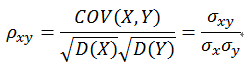称为变量 X 和 Y 的相关系数。若相关系数 = 0，则称 X与Y 不相关。相关系数越大，相关性越大，但肯定小于或者等于1.。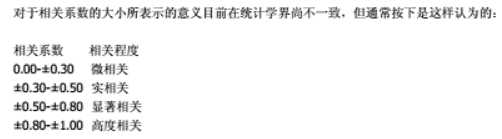相关函数分为自相关互相关

• 自相关函数是描述随机信号 x(t) 在任意不同时刻 t1,t2 的取值之间的相关程度。
• 互相关函数是描述随机信号 x(t)、y(t) 在任意两个不同时刻s，t的取值之间的相关程度

# 2 自相关函数

## 2.1 定义

自相关函数是描述随机信号 x(t) 在任意不同时刻 t1,t2的取值之间的相关程度。自相关函数，是对信号自身的互相关， 表示同一序列不同时刻的相关程度。是用寻找重复模式的数字工具，就如一个存在被覆盖噪声的周期信号，或识别丢失的基频。它经常被用于信号处理中的分析函数或序列，如时域信号 。定义式：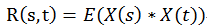或者：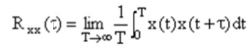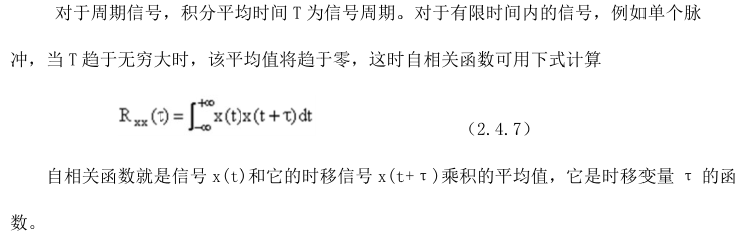## 2.2 性质

主要性质如下：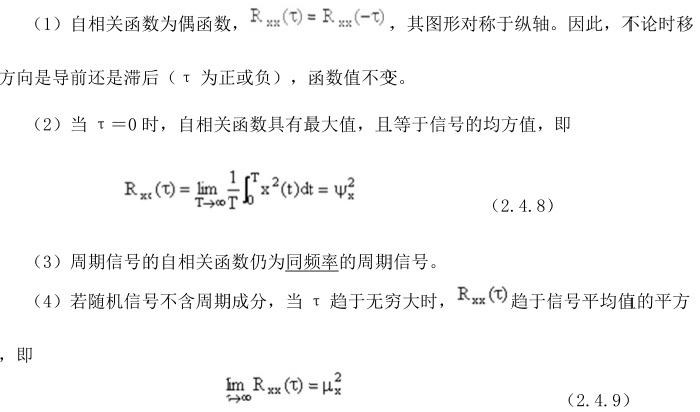自相关系数：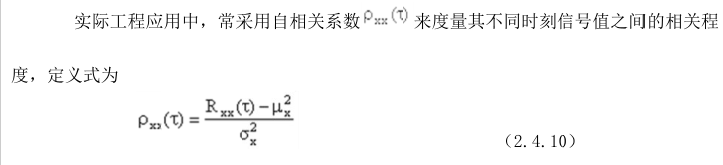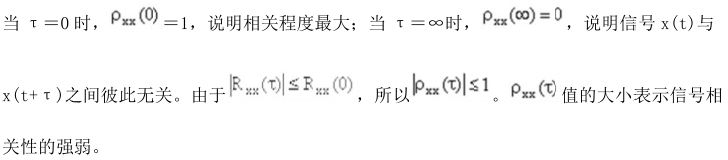# 3 互相关（cross-correlation）函数

## 3.1 定义

自相关是互相关的一种特殊情况.。互相关函数是描述随机信号 x(t)、y(t) 在任意两个不同时刻 s，t 的取值之间的相关程度,其定义为：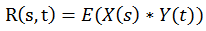对于连续函数，有定义：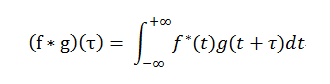对于离散的，有定义：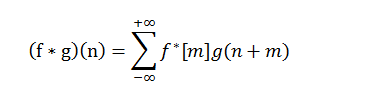从定义式中可以看到，互相关函数卷积运算类似，也是两个序列滑动相乘，但是区别在于：互相关的两个序列都不翻转，直接滑动相乘，求和；卷积的其中一个序列需要先翻转，然后滑动相乘，求和。所以，f(t)g(t) 做相关等于 f*(-t)g(t) 做卷积。

在图象处理中，自相关和互相关函数的定义如下：设原函数是 f(t)，则自相关函数定义为 R(u)=f(t)*f(-t)，其中*表示卷积；设两个函数分别是f(t)和g(t)，则互相关函数定义为R(u)=f(t)*g(-t)，它反映的是两个函数在不同的相对位置上互相匹配的程度。

## 3.2 性质

互相关函数的性质：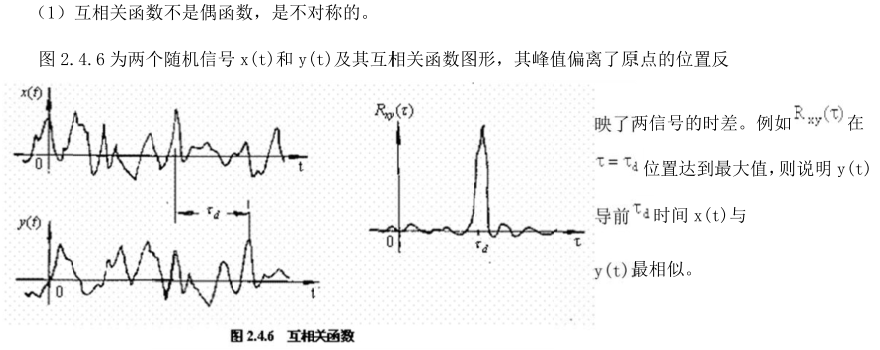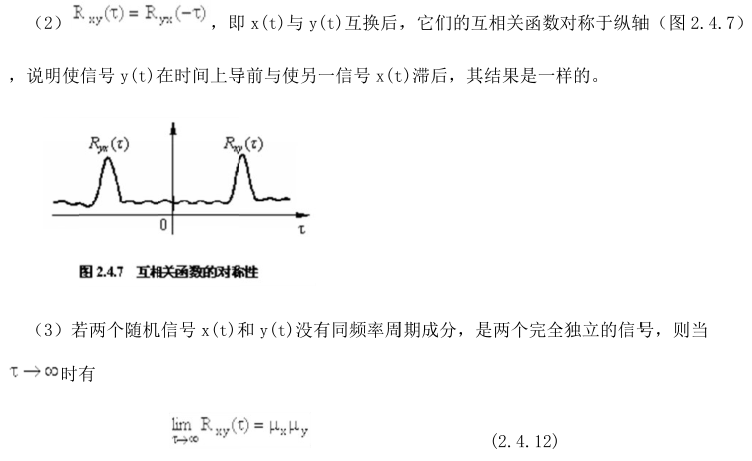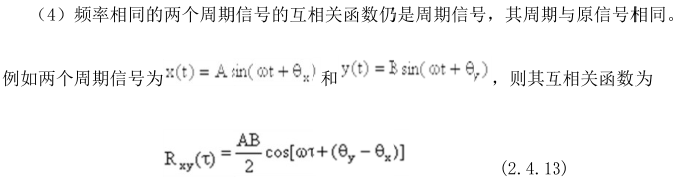互相关系数：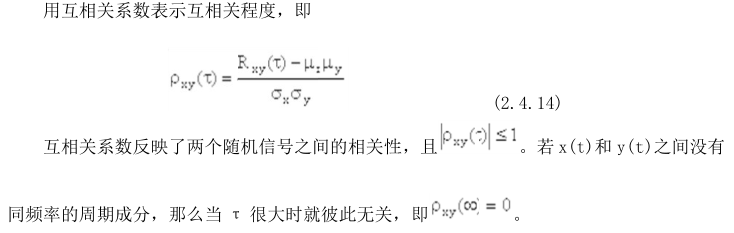正如卷积有线性卷积（linear convolution）循环卷积（circular convolution）之分；互相关也有线性互相关（linear cross-correlation）循环互相关（circular cross-correlation）。线性互相关和循环互相关的基本公式是一致的，不同之处在于如何处理边界数据。其本质的不同在于它们对原始数据的看法不同。

## 3.3 线性互相关（linear cross-correlation）

假设我们手里有两组数据，分别为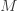个和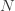个，表示为：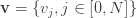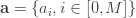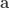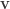长，即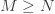。序列之间的线性互相关操作表示为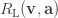，其结果也是一个序列，表示为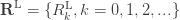。具体的操作是用这两个序列进行的一种类似“滑动点积”的操作，如图1和图2所示。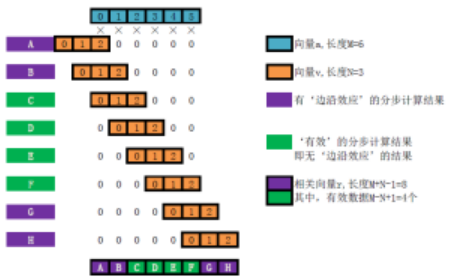图1. 线性互相关的计算过程示意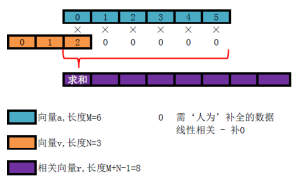图2. 线性互相关结果序列中单个值计算示意

得到的互相关序列总长度为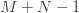，该序列的前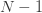和后个数值是无效的，有效的数据共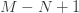个。线性互相关的有效数据第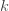个分量的值为：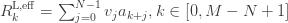注意，线性互相关并不满足交换律，即：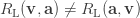一个简单的应证是，等式两侧操作所得结果的有效数据个数都不一致。

线性相关的实际意义是，向量中的各个与向量等长的子向量与向量的相似程度。这样，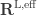中值最大的索引就是与向量中与最相似的子向量的起始索引。通常，为了获得有效的互相关数据，我们总是用较短的数据去滑动点积较长的数据。

用一个实际的应用例子来验证一下吧。如图3的第一个子图表示雷达声纳发射了一个探测信号。经过一段时间之后，收到了如图3的第二个子图所示的回波（带有一定的噪声）。此时我们关注的是如何确定回波中从何时开始是对探测信号的响应，以便计算目标距雷达的距离，这就需要用到线性互相关。在第三个子图中的‘Valid’曲线即是有效互相关数据，其中清晰地呈现出两处与探测信号相似的回波的位置。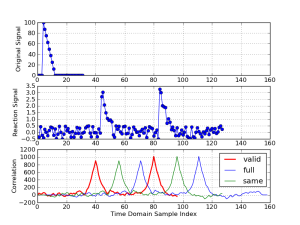图3. 相关计算的一个例子：雷达回波分析

线性互相关中，还有一些概念值得注意：

• 一是补零。由线性相关的计算式不难发现，为了计算出个完整的相关系数序列（包含那些“无效数据”在内的所有结果），需要用到一些“不存在”的点。这就需要人为地对这些值进行补充，在线性相关的计算中，对这些超出原始数据储存的区域取值为零。
• 二是末端效应。由图1可以发现，一头一尾的个互相关数据并没有完全“嵌入”两个原始数组的全部信息，它们或多或少地受到了人为补零的影响。因此一般认为这些数据是不可用的。
• 三是计算模式的选择。这个问题其实是由问题二衍生而来的，就Python语言中的函数而言，至少有两个可以直接计算线性相关：

numpy.correlate(a, v, mode)scipy.signal.correlate(a, v, mode)

它们的调用参数完全相同。在调用时有三种模式可供选择，它们计算的内容是相同的，但是返回值长度各不相同：
mode = ‘valid’：只返回有效的那一部分相关数据，共$M-N+1$个；
mode = ‘same’：只返回与 等长的那一部分相关数据，共$N$个；
mode = ‘full’：返回全部相关数据，共$M+N-1$个。
图3的第三个子图展示了这三种模式的计算结果，在那个例子中，‘valid’模式是最合适的。

## 3.4 循环互相关（Circular Cross-Correlation）的定义和计算

循环互相关是表征两组等长周期性数据之间相似性的操作，其与线性互相关的区别也正由“等长”“周期性”这个两特点产生。在循环互相关中，被处理的原始数据是等长的，即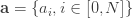。序列之间的线性互相关操作表示为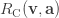，其结果也是一个序列，表示为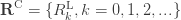。其计算式与线性互相关的写法是一致的：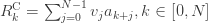只是得到的互相关序列长度也为。循环互相关的计算的具体过程如图4所示，注意到在计算时要用到超出原始数据索引范围的数据，其数据补充方式并不是“补零”而是“周期延拓”：即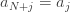。这意味着对于循环互相关，不存在不同的计算模式之分，所有的数据都是有效数据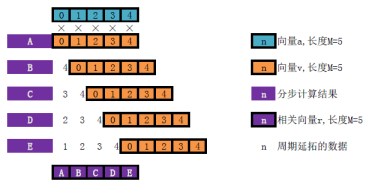图4. 循环互相关的计算过程示意

注意，循环互相关也不满足交换律

这里给出了一个关于循环相关的算例。两路原始数据分别由如下函数生成：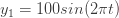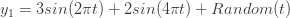如果视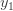为某个线性系统的周期输入信号，而视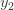为这个线性系统的输出信号。由于存在外接干扰，因此输出信号不完全由输入信号决定。此时，循环互相关的实际意义是，分辨输出信号中的哪一个部分（频率成分）是由该输入信号产生的。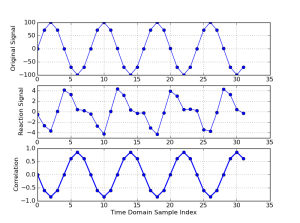图5. 时域数据，从上到下：和他们的循环互相关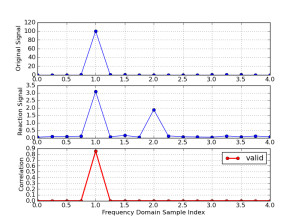图6. 频谱，从上到下：和他们的循环互相关

从图5和图6可以看出，循环互相关的频谱准确地说明了那些测试信号的相关性。

遗憾的是，在Python几大数值计算库中，并没有直接可计算循环相关的函数。但是可以采用如下代码构造出一个可用的（经过归一化的）cxcorr(a, v)函数出来：

def cxcorr(a,v):
nom = np.linalg.norm(a[:])*np.linalg.norm(v[:])
return fftpack.irfft(fftpack.rfft(a)*fftpack.rfft(v[::-1]))/nom

图4中的数据就是通过这个函数计算出来的。其中用到了傅里叶变换反变换来计算循环互相关，这是可行的。它们之间的关系在第四小节的QA中专门讨论。

## 3.5 用线性互相关处理周期性信号

实际上，线性相关也可以处理周期信号，前提是将两组信号采样成长度差异较大的序列。这样，其有效线性互相关也可以完美地反应数据之间的相关性。

同样采用第二节中的例子。这时为了保证足够的有效线性互相关数据，两组数据的长度故意不一致（但都足够表征其特征），如图7所示。它们的频谱如图8所示，仍然完美地体现了测试数据的相关性。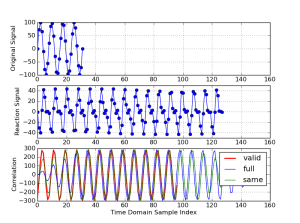图7. 时域数据，从上到下：和他们的线性互相关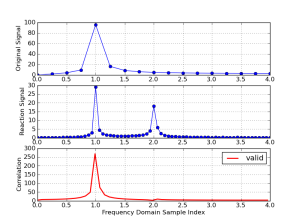图8. 频谱，从上到下：和他们的线性互相关

既然线性互相关也能处理周期性数据，为什么还要专门搞一个基于等长序列和周期延拓的循环互相关呢？实际上，正如后文QA中专门讨论的，这是为了利用快速傅利叶变换加速计算。

## 3.6 相关问题QA

至此，两种常用的互相关评价方法及其计算已经总结完毕。然而其中还有一些细节尚待分辨。例如，序列之间的互相关的计算式：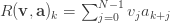与卷积（convolution）的定义式：如此类似，如果再联想起傅里叶变换的卷积定理，那么，至少会产生如下的问题：

Q.1：它们之间有更深意义上的联系吗？
A.1：文献的答复是坚决的：“不要让求卷积和互相关的数学相似性迷惑你，它们描述了不同的信号处理过程。卷积是系统输入信号、输出信号和冲激响应之间的关系互相关是一种在噪声背景下检测已知信号的方法。二者在数学上的相似仅仅是一种巧合。”实际上，只要注意到卷积操作是满足交换律的，而互相关操作并不满足交换律。仅此一点也许就能说明它们有着本质的不同吧。

Q.2：可以利用Python中计算卷积的函数来计算互相关吗？
A.2可以，但是只能用以计算线性互相关。Python中的numpy.convolve()函数就可以计算两个序列之间的卷积。在卷积的计算过程中也会自动进行补零（而不是周期延拓，这就是为什么只能计算线性相关的原因），这种卷积有时被称为线性卷积，同样涉及末端效应、有效数据长度等考虑。具体地，根据相关和卷积的表达式，如果希望计算序列之间的线性互相关序列。等效地，只需要计算序列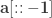之间的卷积。表示序列的“反置”，即将序列[1,2,3]反置为[3,2,1]。

Q.3：可以根据傅立叶变换的性质中有卷积定理，利用傅立叶正/逆变换计算互相关吗？
A.3可以，但是只能用于计算循环互相关。傅立叶变换的卷积定理中所涉及的卷积是循环卷积。与前述的线性卷积是不同的。实际上不同的并不是卷积本身，它们的计算式是一致的，而是在如何看待参与卷积计算的数据，线性卷积认为参与计算的序列之外都是零，而循环卷积认为参与计算的序列是一个无限循环的数据的一段——这导致了它们对“越界”数据的补齐方式不一样。正如线性互相关和循环互相关的区别！先将循环互相关等效为一个循环卷积，再利用快速傅里叶变换计算卷积即可。实际上本文给出的cxcorr(a, v)函数正是利用这一性质来计算循环相关的。其对计算速度的提升是相当明显的。

Q.4：怎样进行归一化（normalization），以便于比较互相关数据？
A.4：根据参考，用公式：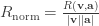## 3.7 参考资料

 Steven W. Smith. Digital Signal Processing: A Practical Guide for Engineering and Scientists [M].
张瑞峰, 詹敏晶 等译. 实用数字信号处理，从原理到应用[M]. 人民邮电出版社, 北京, 2010.
 Mark Owen. Practical Signal Processing [M].
丘天爽, 李丽, 赵林 译. 实用信号处理 [M]. 电子工业出版社, 北京, 2009.
 关于MATLAB中的xcorr() 的论述
http://www.mathworks.cn/cn/help/signal/ref/xcorr.html
 关于MATLAB中的cxcorr() 的论述
http://www.mathworks.com/matlabcentral/fileexchange/4810-circular-cross-correlation
 网络论坛Stackoverflow关于此问题的讨论
http://stackoverflow.com/questions/6991471/computing-cross-correlation-function
http://stackoverflow.com/questions/12323959/fast-cross-correlation-method-in-python
http://stackoverflow.com/questions/9281102/n-fold-fft-convolution-and-circular-overlap
http://stackoverflow.com/questions/6855169/convolution-computations-in-numpy-scipy
http://stackoverflow.com/questions/4688715/find-time-shift-between-two-similar-waveforms
 关于Cross-correlation的定义
http://mathworld.wolfram.com/Cross-Correlation.html
http://paulbourke.net/miscellaneous/correlate/
http://en.wikipedia.org/wiki/Cross-correlation
 关于 Circular Cross-correlation的定义
http://en.wikipedia.org/wiki/Circular_convolution
http://cnx.org/content/m22974/latest/

自相关函数与互相关函数：http://www.doc88.com/p-5129647069822.html

互相关（cross-correlation）及其在Python中的实现：https://blog.csdn.net/icameling/article/details/85238412

展开全文• m序列少值互相关函数一直都是研究者感兴趣的方向之一,但这方面取得的成果并...通过引入矩阵结合方案,把对互相关值分布问题的研究转化为对二次型秩之间关系的研究,最终得出了该类p元m序列之间五值互相关函数的完整分布。
• 相关函数的定义： ...实函数f1(t)f_1(t)f1​(t)与f2(t)f_2(t)f2​(t)，如为能量有限信号，它们之间的互相关函数定义为： R12(τ)=∫−∞∞f1(t)f2(t−τ)dtR_{12}(\tau)=\int_{-\infty}^{\infty}f_1(t)f_2(t-\t

相关函数的定义：
为了比较某信号与另一延时信号 τ \tau 信号之间的相似度，需要引入相关函数的概念。相关函数是鉴别信号的有力工具，被广泛应用于雷达回波的识别，通信同步信号的识别等领域。相关函数也被叫做相关积分，它与卷积的运算方法类似。
实函数 f 1 ( t ) f_1(t) f 2 ( t ) f_2(t) ，如为能量有限信号，它们之间的互相关函数定义为：
R 12 ( τ ) = ∫ − ∞ ∞ f 1 ( t ) f 2 ( t − τ ) d t R_{12}(\tau)=\int_{-\infty}^{\infty}f_1(t)f_2(t-\tau)\rm dt
R 21 ( τ ) = ∫ − ∞ ∞ f 2 ( t ) f 1 ( t − τ ) d t R_{21}(\tau)=\int_{-\infty}^{\infty}f_2(t)f_1(t-\tau)\rm dt

一般情况下 R 12 ( τ ) ! = R 21 ( τ ) R_{12}(\tau)!=R_{21}(\tau) ，一般 R 12 ( τ ) = − R 21 ( τ ) R_{12}(\tau)=-R_{21}(\tau)

如果 f 1 ( t ) f_1(t) f 2 ( t ) f_2(t) 是同一信号，可记为 f ( t ) f(t) ,这个时候无需区分 R 12 , R 21 R_{12},R_{21} 而是使用自相关函数 R ( τ ) R(\tau) 表示。

R ( τ ) = ∫ − ∞ ∞ f ( t ) f ( t − τ ) d t R(\tau)=\int _{-\infty}^{\infty}f(t)f(t-\tau) \rm dt

可以看出，对自相关函数有:
R ( τ ) = R ( − τ ) R(\tau)=R(-\tau)
可见，实函数f(t)的自相关函数是时移 τ \tau 的偶函数。

展开全文• ## 互相关函数的计算

千次阅读 2021-10-19 13:30:14
目录互相关函数的定义互相关函数的计算存在具体项目参数时 整理不易，有条件的点个关注、点个赞呗！感恩各位大哥！ 互相关函数的定义 描述两个不同的信号在不同时期上的相关性的函数，主要应用： 混有周期成分数据...

# 互相关函数的定义

描述两个不同的信号在不同时期上的相关性的函数，主要应用：

• 混有周期成分数据（信号）的频率（周期）提取，例如两列数据在其中一列数据滞后三期时相关性最高，则该类数据的周期为3。

# 互相关函数的计算

公式：
C 1 , 2 ( t ) ≈ ∫ 0 t c v 1 ( τ ) v 2 ( t + τ ) d τ (1) C_{1,2}(t)\approx\int_{0}^{t_{c}}v_{1}(\tau)v_{2}(t+\tau)d\tau\tag{1}
实际计算举例，本实验中主要针对采用傅里叶变换的循环互相关，因此后续以循环互相关为例进行讲解！
循环互相关的思想如下：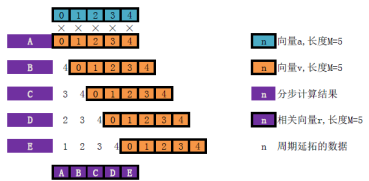注意到在计算时要用到超出原始数据索引范围的数据，其数据补充方式并不是“补零”而是“周期延拓”。
循环互相关实际则通过傅里叶变换进行计算，将上述两列数据分别变换到频率域，从而得到频率域同等长度的两列数据 [ 10. + 0. j − 2.5 + 3.4409548 j − 2.5 + 0.81229924 j ] ， [ 10. + 0. j − 2.5 + 3.4409548 j − 2.5 + 0.81229924 j ] [10. +0.j -2.5+3.4409548j -2.5+0.81229924j]，[10. +0.j -2.5+3.4409548j -2.5+0.81229924j] ，则输出互相关矩阵为第一个元素为：
( 10 ∗ 10 + 0 j ∗ 0 j ) + ( 10 ∗ 0 j − 0 j ∗ 10 ) = ( 100 + 0 j ) (10*10+0j*0j)+(10*0j-0j*10)=(100+0j) ，同理第二个元素为 （ − 2.5 ∗ − 2.5 + 3.4409548 j ∗ 3.4409548 j ） + （ − 2.5 ∗ 3.4409548 j + 2.5 ∗ 3.4409548 j ) （-2.5*-2.5+3.4409548j *3.4409548j ）+（-2.5*3.4409548j+2.5*3.4409548j)

# 存在具体项目参数时

计算方式一：

            for c in range(len(irover)):
# ================= Non center Station Index =================
Atr=st[irover[c]]
# ================= Location =================
# ================= Do FFT at non center =================
freq_rover, time_rover, Sxx_rover_ = sg.spectrogram(Atr.data  ,
nperseg=wd, # 窗口大小，对应fftlen
noverlap=0, # noverlap对应overlaprate，但是noverlap是具体值，overlaprate是重叠率
nfft=nft, # 实际就是窗口大小，表示是否对数据进行填充。
fs=Fs, # 频率，暂时不清楚频率在计算中的作用
scaling='spectrum', # 输出设置为功率谱
mode='complex')

# ======== Find Data in range output frequency ===========
Sxx_rover_=Sxx_rover_[idx]

# ================= Measure AutoCorrelation Ratio ================
rat_autocorr=np.zeros((len(Sxx_pusat_[:,0]),len(Sxx_pusat_[0,:])), dtype=np.complex)
for nwd in range(len(Sxx_pusat_[0,:])):
Abs_Sxx_pusat=(abs(Sxx_pusat_[:,nwd]))
Smooth_Abs_Sxx_pusat=sg.convolve(Abs_Sxx_pusat,smooth,mode='same')

Abs_Sxx_rover=(abs(Sxx_rover_[:,nwd]))
Smooth_Abs_Sxx_rover=sg.convolve(Abs_Sxx_rover,smooth,mode='same')

rat_autocorr[:,nwd]=((Sxx_pusat_[:,nwd]*np.conj(Sxx_rover_[:,nwd]))/
(Smooth_Abs_Sxx_pusat*Smooth_Abs_Sxx_rover))

time_autocorr=np.mean(rat_autocorr,axis=1)
autocorr[:,c]=time_autocorr


计算方式二：

for(long i=0;i<d.nsta;i++){
if(((k*d.steplen)>=d.startend[i*2])&&((k*d.steplen+d.fftlen)<d.startend[i*2+1]))
{
ifCC[i] = 1;
}
else
{
ifCC[i] = 0;
}

if (ifCC[i]){
for(long j=0;j<d.fftlen;j++){
in[i][j] = d.data[j+k*d.steplen+d.npts*i];
}
fftwf_execute(p[i]);
if(d.ifspecwhitenning){
for (long j=0;j<d.nf;j++){
absv = sqrtf(out[i][j*d.fstride]*out[i][j*d.fstride]+out[i][j*d.fstride]*out[i][j*d.fstride]);
absv = max(absv,1e-8f);
if(absv!=0){
datar[j+d.nf*i] = out[i][j*d.fstride]/absv;
datai[j+d.nf*i] = out[i][j*d.fstride]/absv;
}
}
}
else{
for(long j=0;j<d.nf;j++){
datar[j+d.nf*i] = out[i][j*d.fstride];
datai[j+d.nf*i] = out[i][j*d.fstride];
}
}
}
}
#ifdef useOMP
#pragma omp parallel
#pragma omp for
#endif
for(long i=0;i<npairs; i++){
if(ifCC[d.Pairs[i*2]]&ifCC[d.Pairs[i*2+1]]){
for(long j=0;j<d.nf;j++){
d.ncfsr[j+i*d.nf] += datar[j+d.Pairs[i*2]*d.nf]*datar[j+d.Pairs[i*2+1]*d.nf] + datai[j+d.Pairs[i*2]*d.nf]*datai[j+d.Pairs[i*2+1]*d.nf];
d.ncfsi[j+i*d.nf] += datar[j+d.Pairs[i*2]*d.nf]*datai[j+d.Pairs[i*2+1]*d.nf] - datai[j+d.Pairs[i*2]*d.nf]*datar[j+d.Pairs[i*2+1]*d.nf];
}
CCnumbers[i] = CCnumbers[i] + 1;
}
}
}


相同之处：均采用傅里叶变换求解
不同之处：方法二窗口的指定是在傅里叶变换函数包的外面，且其将数据集切分为每步再传入傅里叶变换函数。方法一在傅里叶变换中指定数据窗口大小和每次移动步长，暂时不知道这两种方式是否具有差异。

展开全文互相关计算
•能量信号 互相关函数
• 一、功率信号的互相关函数、 二、功率信号的自相关函数互相关函数
• 互相关函数是在频域内两个信号是否相关的一个判断指标，把两测点之间信号的互谱与各自的自谱联系了起来。它能用来确定输出信号有多大程度来自输入信号，对修正测量中接入噪声源而产生的误差非常...
• 自相关函数 互相关函数自协方差矩阵 互协方差矩阵的区别联系 : https://mermaidjs.github.io/ : http://adrai.github.io/flowchart.js/
• %-----------------------------------------------------------------% exa011007_xcorr.m: for example 1.10.7 and example 1.8.3% to test xcorr.m% 求两个序列的互相关函数，或一个序列的自相关函数；...
• 互相关函数、互功率谱和卷积之间的关系 1.我们要实现怎样的目标？ 如果有两个复信号， 连续信号表示为y1(t)y_1(t)y1​(t)和 y2(t)y_2(t)y2​(t); 离散信号表示为y1(n)y_1(n)y1​(n)和y2(n)y_2(n)y2​(n)； 两个信号...
• 零均值归一化互相关函数是用于对参考子区和目标子区进行相关性测量，当值为1时，相关性最好，下面是零均值归一化互相关函数的公式： 话不多说，上代码，下面是MATLAB代码实现：该脚本操作很简单，将代码复制到...MATLAB代码
• 零均值归一化互相关函数相对于别的一些相关函数，精度虽然没有多大提升，但是它具有较强的抗干扰性和鲁棒性。所以，在数字图像相关法（DIC）的图像匹配过程中，一般都是使用零均值归一化互相关函数，下面是其公式： ...
• ## 互相关函数的频域计算

万次阅读 多人点赞 2018-04-07 14:00:31
互相关函数的频域计算 1.时域计算 x1(n)与x2(n)的互相关定义如下x1(n)与x2(n)的互相关定义如下x_1(n)与x_2(n)的互相关定义如下 R(τ)=E[x1(m)x2(m+τ)]R...
• ## 自相关、互相关函数学习笔记

千次阅读 多人点赞 2019-07-17 15:56:50
为什么相关性有效 参考文献 【1】自相关的物理意义 【2】自相关和互相关函数计算方法总结及心得体会笔记
• 假设两个平稳信号 $\textbf{x}$ 和 $\textbf{y}$ ，如果 $x\left(t+\tau\right)= y\left(t\right)$ ，则可通过互相关求 \$\...XCORR 实现首先，通过实现 xcorr 函数介绍互相关计算流程：clcclearclose% 实现 xcorr 函...
• 文章参考：https://blog.csdn.net/wordwarwordwar/article/details/63253272  ... 相关函数定义：随机序列的不同时刻的状态之间，存在着关联性或者说不同时刻的状态之间相互有影响，包括随机序列本身...
• 2. 关于自相关函数和互相关函数的Matlab计算。 3. 关于小m序列和Gold序列的一些基本概念 一、学工的如果不知道如何使用DFT来求线性卷积和这种思想的重要性，他就白学了（小波老师原话） 要求：t[n]=f(n)*g(n),...
• 1. 首先说说自相关和互相关的概念。  这个是信号分析里的概念，他们分别表示的是两个时间序列之间和同一个时间序列在任意两个不同时刻的取值之间的相关程度，即互相关 函数是描述随机信号x(t),y(t)在任意两个...偏自相关 自协方差
• 定义了一个函数用来计算两个序列的互相关值，根据给定的两个序列计算其互相关值并以图形方式输出结果。
• ## 互相关函数Rxy(C)

千次阅读 2021-04-22 12:00:55
互相关函数Rxy(力表示一随机振TM1616动信号x(t)在f时刻的值和另一随机振动信号y(t)在(f+T)时刻的值乘积的平均值，它与自相关函数一样，同样是时移的函数。它表示了两个随机振动信号之间的依赖性。互相关函数的数学...
• 2. 关于自相关函数和互相关函数的Matlab计算。3. 关于小m序列和Gold序列的一些基本概念一、学工的如果不知道如何使用DFT来求线性卷积和这种思想的重要性，他就白学了(小波老师原话)要求：t[n]=f(n)*g(n),把g(n)翻转...
• 互相关函数是在频域内两个信号是否相关的一个判断指标，把两测点之间信号的互谱与各自的自谱联系了起来。它能用来确定输出信号有多大程度来自输入信号，对修正测量中接入噪声源而产生的误差非常...
• 信号x（n）=sin(2*pi*0.05*n)+2*cos(2*pi*0.12*n)+w（n），求N=365的相关函数和功率谱
• 在学习概率统计之前，我学习的都是确定的函数。概率统计讨论了一次取值时获得的值是不确定的，而随机过程讨论了不确定会发生哪个时间函数。 每个x(t)函数(样本函数)就是实际发生的一个表达式确定的函数，...自相关函数协方差 机器学习
• 互相关函数 信号y(n)延迟m个时间间隔后与x(n)的互相关函数定义如下： 此定义可以理解为x(n)不动，y(n)左移m个时间单位后与x(n)对应相乘求和的结果。 调换x和y的位置，可得： 理解其几何意义： ：y(n)不动...现代信号处理...